# Meta-theory

(diff) ← Older revision | Latest revision (diff) | Newer revision → (diff)

The totality of mathematical methods and means intended for the description and definition of some formal axiomatic theory, as well as for the investigation of its means. A meta-theory is an important component of the formalization method, which is one of the most central methods in mathematical logic.

The essence of this method may briefly be described as follows. Suppose that one is interested in some meaningful mathematical theory. This may be a complicated theory, the semantics of which is intuitively not sufficiently clear (e.g. it may be set theory, mathematical analysis, second-order arithmetic, etc.). One is interested in whether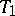is non-contradictory or whether some mathematical principle (e.g. the axiom of choice) is compatible with. In order to clarify this question one constructs at first a precise logico-mathematical languagesuch that all statements inof interest can be described by formulas in. Then the logical principles that are used in the theory to derive new facts are formalized as axioms and derivation rules; these are used to derive new formulas in the languagefrom the axioms and formulas already derived. Thus, a formal system (or, in other words, a formal axiomatic theory, a calculus)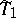arises that exactly describes some fragment of the meaningful theoryof interest. It is essential here that in statingone does not use an exhaustive penetration into the, possibly very complicated, semantics of. The calculusis constructed by simple laws as a purely symbolic system and for understanding its operation one need not penetrate into the sense of the formulas that are derived in it.

Such an approach opens, first, the possibility of mathematically formulating the problems of interest, related to the derivability of certain formulas in, and, secondly, of investigatingby means of some meaningful theory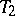. In this case,is called the object theory, andits meta-theory.

From the point of view of the foundations of mathematics it is important thatis related to more reliable theories than, so that investigatingby means ofcan be regarded as a real clarification and justification of the unclear part of the semantics ofusing the more convincing theory. In this connection special preference has been given to sufficiently reliable meta-theories reflecting finite parts of mathematics, as well as to theorems constructed within the frame of intuitionism or constructive mathematics. Moreover, outside the foundations of mathematics this restriction is superfluous. If one is interested not in the question of intuitive clarity ofbut only in the simple fact of derivability or non-derivability of certain formulas in, it is natural to investigateby means of any previously established mathematical theorythat is convincing for the investigator, without imposing a priori restrictions.

One may further analogously investigate the meta-theory, by constructing a formal system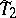and by studyingby means of some meta-meta-theory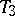. The investigations in proof theory are of this nature.

How to Cite This Entry:
Meta-theory. Encyclopedia of Mathematics. URL: http://encyclopediaofmath.org/index.php?title=Meta-theory&oldid=16348
This article was adapted from an original article by A.G. Dragalin (originator), which appeared in Encyclopedia of Mathematics - ISBN 1402006098. See original article